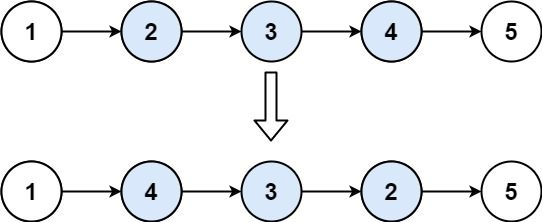1. 反转链表 II

2. 解码方法

3. 擅长编码的小k

???? 每日一练刷题专栏

Golang每日一练 专栏

Python每日一练 专栏

C/C++每日一练 专栏

Java每日一练 专栏

# 1. 反转链表 II```输入：head = [1,2,3,4,5], left = 2, right = 4

```输入：head = , left = 1, right = 1

```

• 链表中节点数目为 n
• 1 <= n <= 500
• -500 <= Node.val <= 500
• 1 <= left <= right <= n

https://edu.csdn.net/practice/23155429

1. #include <stdio.h>
2. #include <stdlib.h>
3. struct ListNode
4. {
5. int val;
6. struct ListNode *next;
7. };
8. static struct ListNode *reverseBetween(struct ListNode *head, int m, int n)
9. {
10. int i;
11. struct ListNode dummy;
12. struct ListNode *prev = &dummy;
14. for (i = 1; i < m; i++)
15. {
16. prev = prev->next;
17. }
18. struct ListNode *p = prev->next;
19. for (i = m; i < n; i++)
20. {
21. struct ListNode *q = p->next;
22. p->next = q->next;
23. q->next = prev->next;
24. prev->next = q;
25. }
26. return dummy.next;
27. }
28. int main()
29. {
30. struct ListNode dummy;
31. struct ListNode *prev = &dummy;
32. struct ListNode *p;
33. int arr[] = {1,2,3,4,5};
34. int count = sizeof(arr)/sizeof(arr);
35. for (int i = 0; i < count; i++)
36. {
37. p = (ListNode*)malloc(sizeof(*p));
38. p->val = arr[i];
39. p->next = NULL;
40. prev->next = p;
41. prev = p;
42. }
43. int m = 2;
44. int n = 4;
45. struct ListNode *head = reverseBetween(dummy.next, m, n);
46. for (p = head; p != NULL; p = p->next)
47. {
48. printf(“%d “, p->val);
49. }
50. printf(“\n”);
51. return 0;
52. }1 4 3 2 5

# 2. 解码方法

`'A' -> 1'B' -> 2...'Z' -> 26`

• “AAJF” ，将消息分组为 (1 1 10 6)
• “KJF” ，将消息分组为 (11 10 6)

```输入：s = "12"

```输入：s = "226"

```输入：s = "0"

```输入：s = "06"

```

• 1 <= s.length <= 100
• s 只包含数字，并且可能包含前导零。

https://edu.csdn.net/practice/23155430

1. #include <stdio.h>
2. #include <stdlib.h>
3. #include <string.h>
4. static int numDecodings(char *s)
5. {
6. int len = strlen(s);
7. if (len == 0)
8. {
9. return 0;
10. }
11. int a = 1;
12. int b = s == ‘0’ ? 0 : a;
13. int c = b;
14. for (int i = 2; i <= len; i++)
15. {
16. c = s[i – 1] == ‘0’ ? 0 : b;
17. int num = (s[i – 2] – ‘0’) * 10 + (s[i – 1] – ‘0’);
18. if (num >= 10 && num <= 26)
19. {
20. c += a;
21. }
22. a = b;
23. b = c;
24. }
25. return c;
26. }
27. int main()
28. {
29. printf(“%d\n”, numDecodings((char*)”12″));
30. printf(“%d\n”, numDecodings((char*)”226″));
31. printf(“%d\n”, numDecodings((char*)”0″));
32. printf(“%d\n”, numDecodings((char*)”06″));
33. return 0;
34. }2
3
0
0

# 3. 擅长编码的小k

`orthography`

`gtorhoprahy`

100%的数据，字符串长度不超过20000

https://edu.csdn.net/practice/23155431

1. #include <iostream>
2. #include <cstring>
3. using namespace std;
4. void shuchu(char *a, int m, int n)
5. {
6. if (n <= 0 || m <= 0 || m > n)
7. {
8. return;
9. }
10. else
11. {
12. cout << a[(m + n) / 2];
13. shuchu(a, m, (m + n) / 2 – 1);
14. shuchu(a, (m + n) / 2 + 1, n);
15. }
16. }
17. int main()
18. {
19. char a;
20. char b;
21. cin >> a;
22. for (int i = 0; i < 20000; i++)
23. {
24. b[i + 1] = a[i];
25. }
26. int n = strlen(a);
27. shuchu(b, 1, n);
28. return 0;
29. }orthography
gtorhoprahy

# 每日一练刷题专栏

✨ 持续，努力奋斗做强刷题搬运工！

???? 点赞，你的认可是我坚持的动力！

???? 收藏，你的青睐是我努力的方向！

✎ 评论，你的意见是我进步的财富！## Golang每日一练 专栏## Python每日一练 专栏## C/C++每日一练 专栏